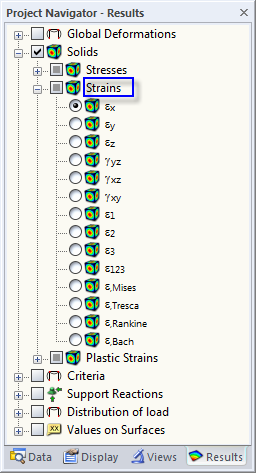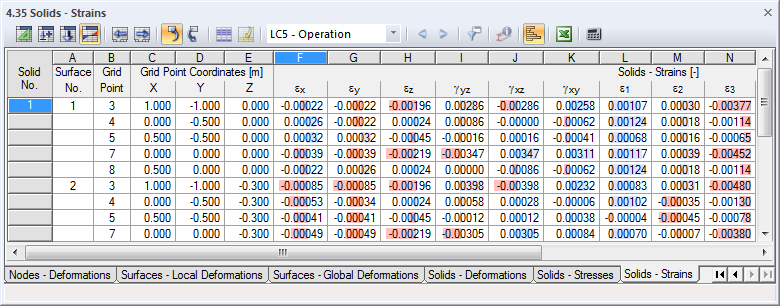# RFEM

Online manuals, introductory examples, tutorials, and other documentation.

# 8.35 Solids - Strains

The general definition of the tensor for the spatial strain state is as follows:

The individual elements of the tensor are defined as follows:

To control the graphical display of solid strains, select Solids → Strains in the Results navigator. Table 4.35 shows these strains in numerical form.Image 8.72 Results navigator: Solids → StrainsImage 8.73 Table 4.35 Solids - Strains

The table shows the strains sorted by surfaces. The results are listed in reference to the grid points of each surface that encloses the solid.

The Grid Point and Grid Point Coordinates table columns correspond to those of the previous results table 4.34 Solids - Stresses.

Solids - Strains

The strains are directly determined from the eigenvalues of the strain matrix by the analysis core. When the model is analyzed according to the linear static or second-order analysis, a linear calculation is performed; for a calculation according to the large deformation analysis, strains are determined with a logarithmic approach.

The equivalent strains are determined according to the four stress hypotheses as follows:

 εMises εTresca Maximum of the eigenvalue differences according to the matrix R (see Equation 8.17) εRankine Maximum of the eigenvalues according to the matrix R εBach Maximum of the eigenvalue differences by considering Poisson's ratio ν according to the matrix R